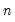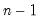Command Reference : Operator and Function Reference : By-Group Statistics

By-Group Statistics
The following “by group”-statistics are available in EViews. They may be used as part of a series expression statements to compute the statistic for subgroups, and to assign the value of the relevant statistic to each observation.
The functions take a series expression arg1 for which we wish to compute group statistics and one or more series expressions arg2, defining the groups over which we wish to compute the statistics. In the leading case, you will provide a single series expression containing categories. More generally, you can provide several series expressions separated by commas (arg2, arg3, ...) that jointly define the categories. For brevity, the table entries below only depict a single categorical variable arg2.

 Function Description @obsby(arg1, arg2[, s]) Number of non-NA arg1 observations for each arg2 group. @nasby(arg1, arg2[, s]) Number of arg1 NA values for each arg2 group. @sumsby(arg1, arg2[, s]) Sum of arg1 observations for each arg2 group. @meansby(arg1, arg2[, s]) Mean of arg1 observations for each arg2 group. @minsby(arg1, arg2[, s]) Minimum value of arg1 observations for each arg2 group. @maxsby(arg1, arg2[, s]) Maximum value of arg1 observations for each arg2 group. @mediansby(arg1, arg2[, s]) Median of arg1 observations for each arg2 group. @varsby(arg1, arg2[, s]) Variance of arg1 observations for each arg2 group (division by). @varssby(arg1, arg2[, s]) Sample variance of arg1 observations for each arg2 group (division by). @varpsby(arg1, arg2[, s]) Variance of arg1 observations for each arg2 group (division by). @stdevsby(arg1, arg2[, s]) Sample standard deviation of arg1 observations for each arg2 group (division by). @stdevssby(arg1, arg2[, s]) Sample standard deviation of arg1 observations for each arg2 group (division by). @stdevpsby(arg1, arg2[, s]) Standard deviation of arg1 observations for each arg2 group (division by). @sumsqsby(arg1, arg2[, s]) Sum of squares of arg1 observations for each arg2 group. @quantilesby(arg1, arg2, arg3[, s]) Quantiles of arg1 observations for each arg2 group where arg1 is the desired quantile. @skewsby(arg1, arg2[, s]) Skewness of arg1 observations for each arg2 group. @kurtsby(arg1, arg2[, s]) Kurtosis of arg1 observations for each arg2 group. @firstsby(arg1, arg2[, s]) First non-missing value in arg1 for each arg2 group @lastsby(arg1, arg2[, s]) Last non-missing value in arg1 for each arg2 group
With the exception of @quantileby, all of the functions take the form:
@STATBY(arg1, arg2[, arg3, arg4, ..., argn, s])
where @STATBY is one of the by-group function keyword names, arg1 is a series expression, arg2 and the optional arg3 to argn are numeric or alpha series expression identifying the subgroups, and s is an optional sample literal (a quoted sample expression) or a named sample. With the exception of @obsby, arg1 must be a numeric series.
By default, EViews will use the workfile sample when computing the descriptive statistics. You may provide the optional sample s as a literal (quoted) sample expression or a named sample.
The @quantileby function requires an additional argument for the quantile value that you wish to compute:
@quantileby(arg1, arg2[, arg3, arg4, ..., argn], q[, s])
For example, to compute the first quartile, you should use the q value of 0.25.
There are two related functions of note,
@groupid(arg1[, arg2, arg3, arg4, ..., argn, smpl]])
returns an integer indexing the group ID for each observation of the series or alpha expression arg.